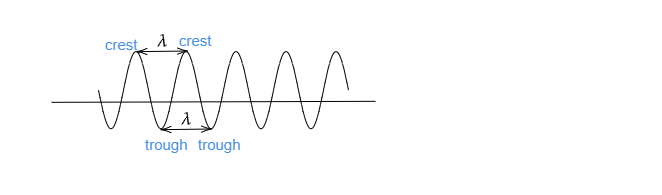# What is wavelength?

Wavelength: The distance between two successive crests or two successive troughs in a wave is known as the wavelength. It is denoted by $\lambda$. Its SI unit is $meter$.Wavelength $\lambda=\frac{v}{f}$

Where $v\rightarrow$ velocity

$f\rightarrow$frequency

Updated on: 10-Oct-2022

52 Views